• Slides: 482 x Quadratic Equations have (or some variable, squared) in them and are equations. x 2 + 5 x + 6 = 0 2 n – 7 n = 18 2 2 x = 11 x + 40 p 2 = 16 (x + 7)(x + 2) = 0The key to solving quadratic equations is the 0 property of multiplication If the product of two quantities is 0, then one of those quantities must be 0.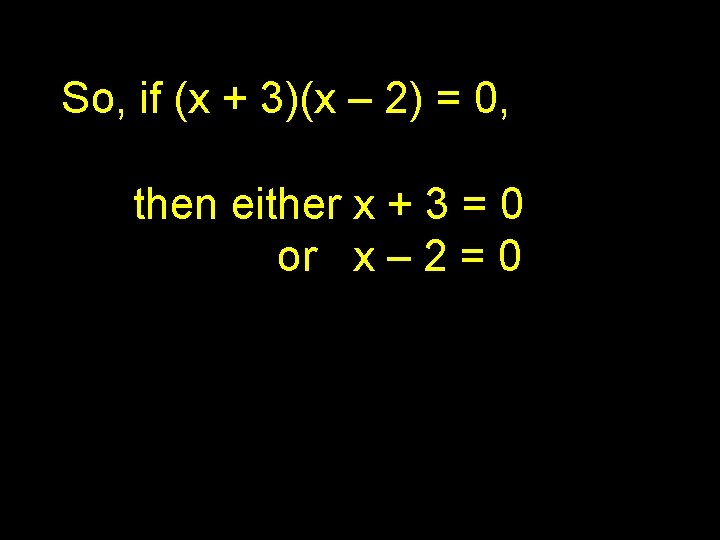So, if (x + 3)(x – 2) = 0, then either x + 3 = 0 or x – 2 = 0So, if (x + 3)(x – 2) = 0, then either x + 3 = 0 or x – 2 = 0 This means either x = -3 or x = 2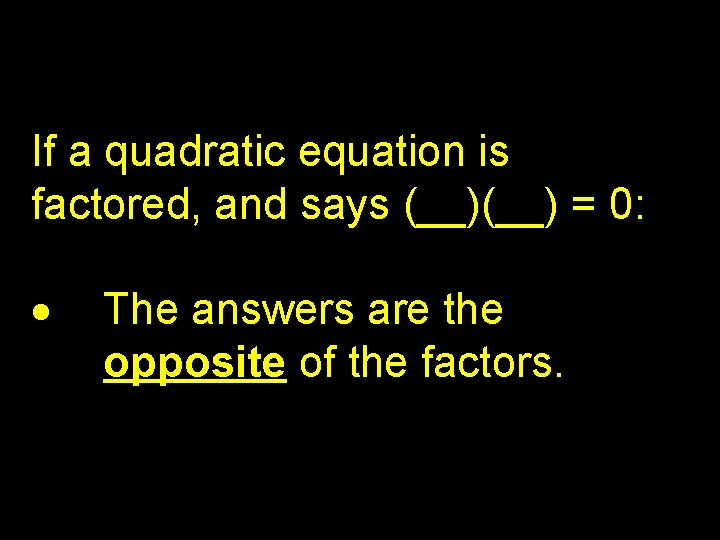If a quadratic equation is factored, and says (__) = 0: The answers are the opposite of the factors.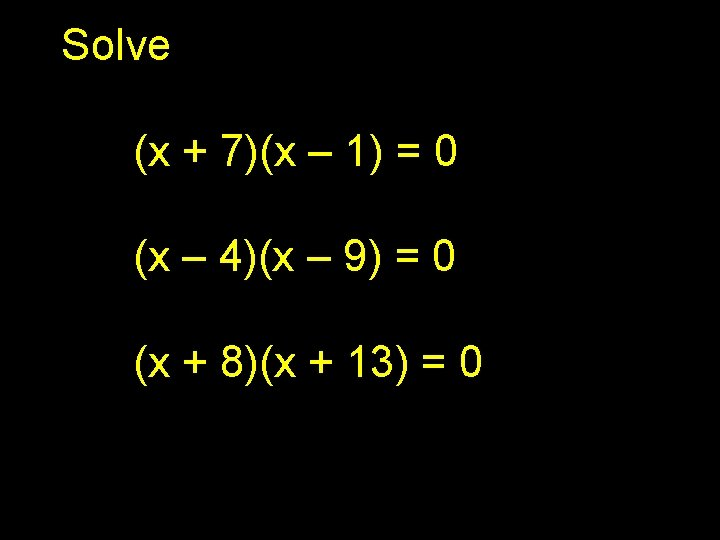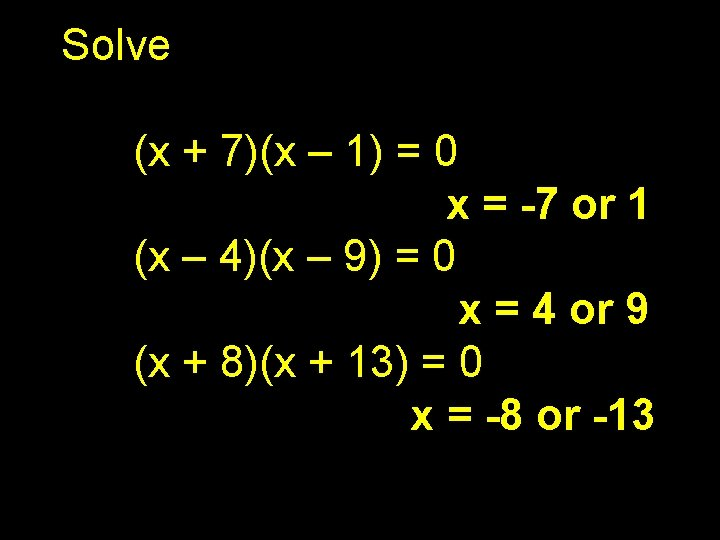Solve (x + 7)(x – 1) = 0 x = -7 or 1 (x – 4)(x – 9) = 0 x = 4 or 9 (x + 8)(x + 13) = 0 x = -8 or -13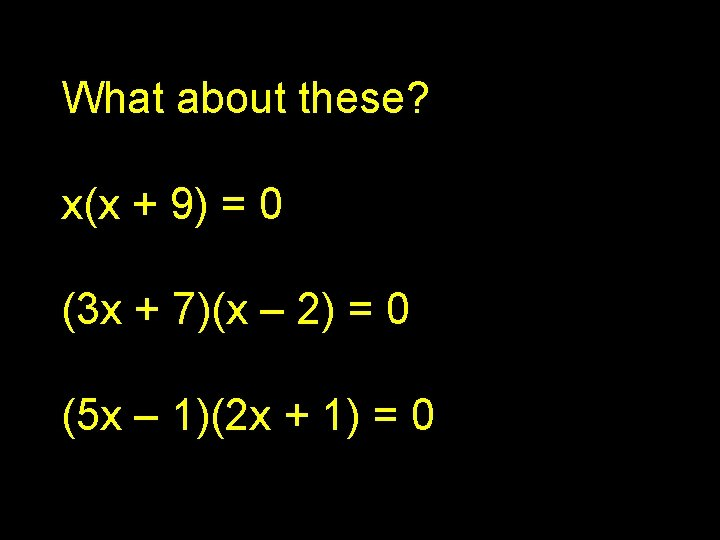What about these? x(x + 9) = 0 (3 x + 7)(x – 2) = 0 (5 x – 1)(2 x + 1) = 0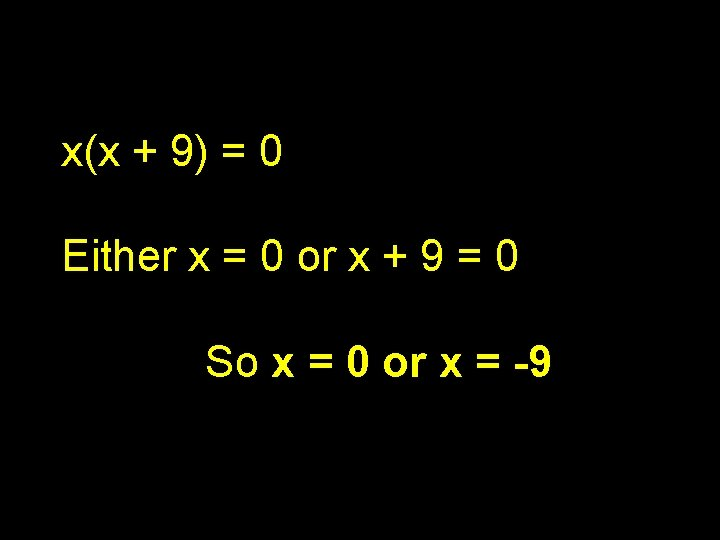x(x + 9) = 0 Either x = 0 or x + 9 = 0 So x = 0 or x = -9(3 x + 7)(x – 2) = 0 Either 3 x + 7 = 0 or x – 2 = 0 So x= -7/ 3 or x = 2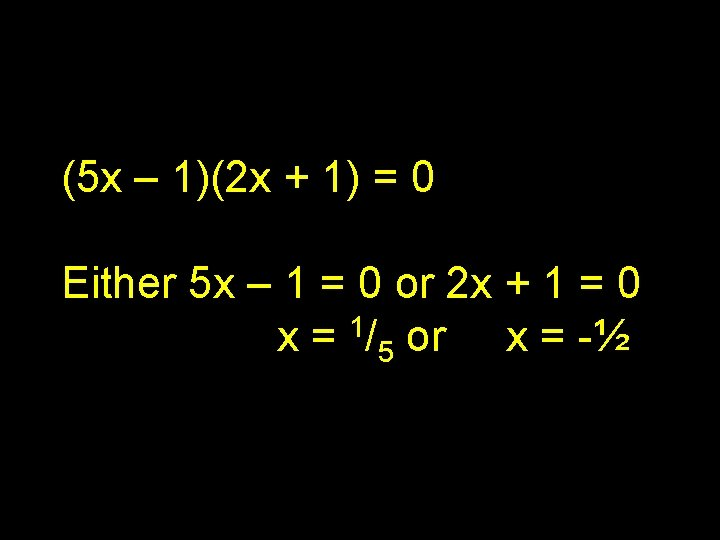(5 x – 1)(2 x + 1) = 0 Either 5 x – 1 = 0 or 2 x + 1 = 0 x = 1/5 or x = -½When there are coefficients, you can find the answers quickly by making a fraction, reading the factor backwards. (9 x – 13)(2 x + 7) = 0 x= 13/ 9 or x = -7/ 2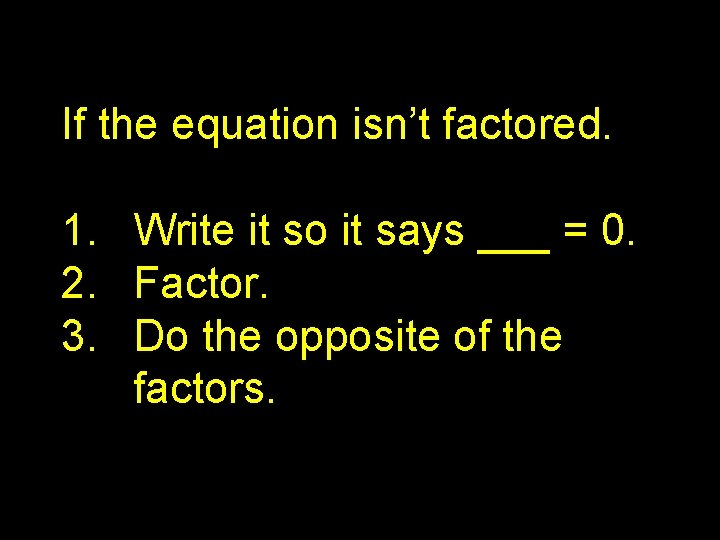If the equation isn’t factored. 1. Write it so it says ___ = 0. 2. Factor. 3. Do the opposite of the factors.n 2 – 7 n = 18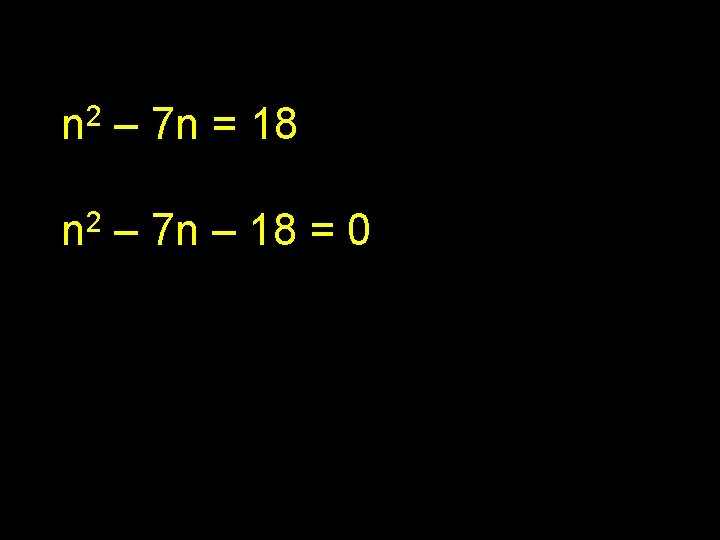n 2 – 7 n = 18 2 n – 7 n – 18 = 0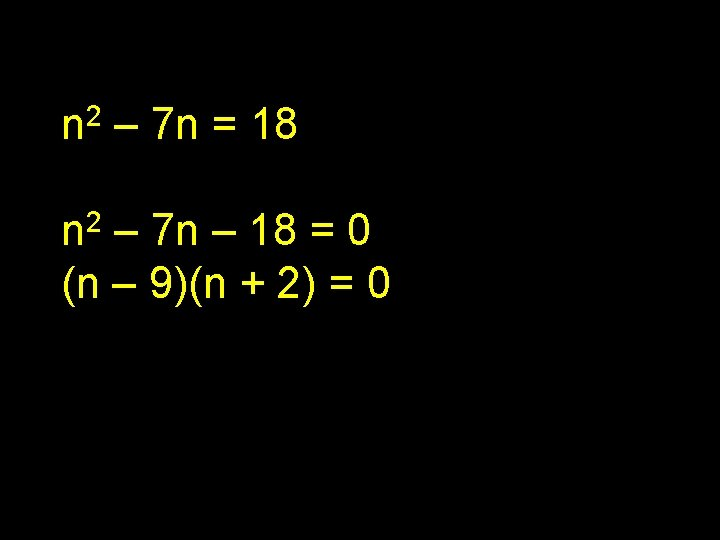n 2 – 7 n = 18 2 n – 7 n – 18 = 0 (n – 9)(n + 2) = 0 n = 9 or -2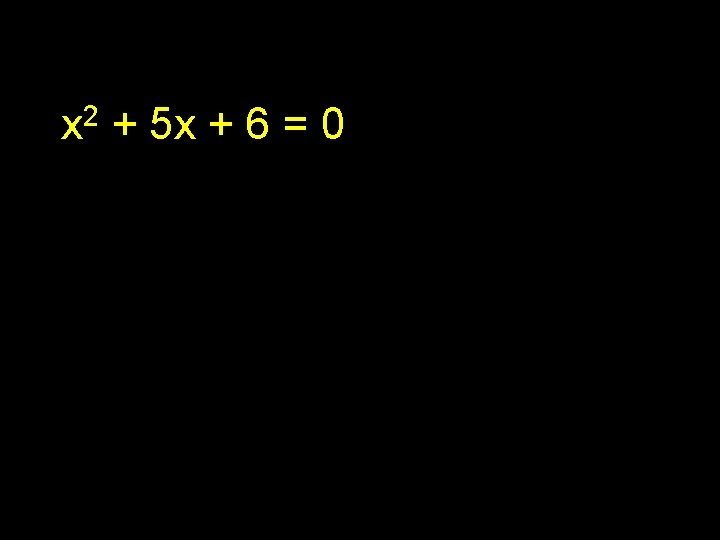x 2 + 5 x + 6 = 0x 2 + 5 x + 6 = 0 Already says __ = 0 (x + 2)(x + 3) = 0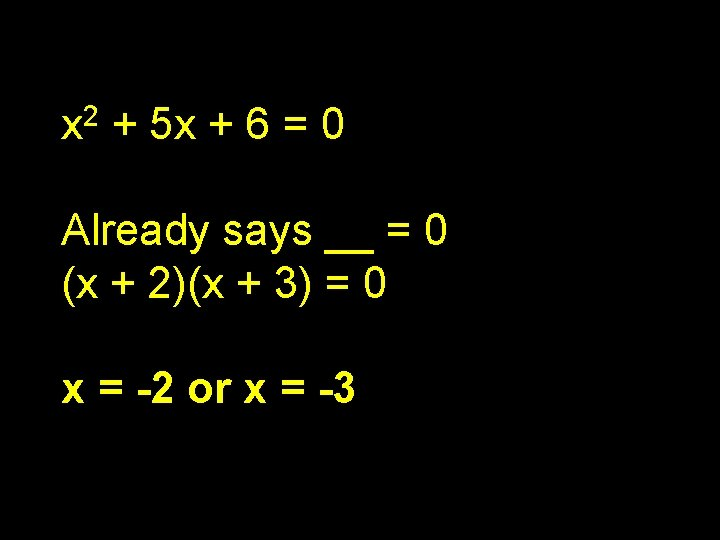x 2 + 5 x + 6 = 0 Already says __ = 0 (x + 2)(x + 3) = 0 x = -2 or x = -32 x 2 = 11 x + 40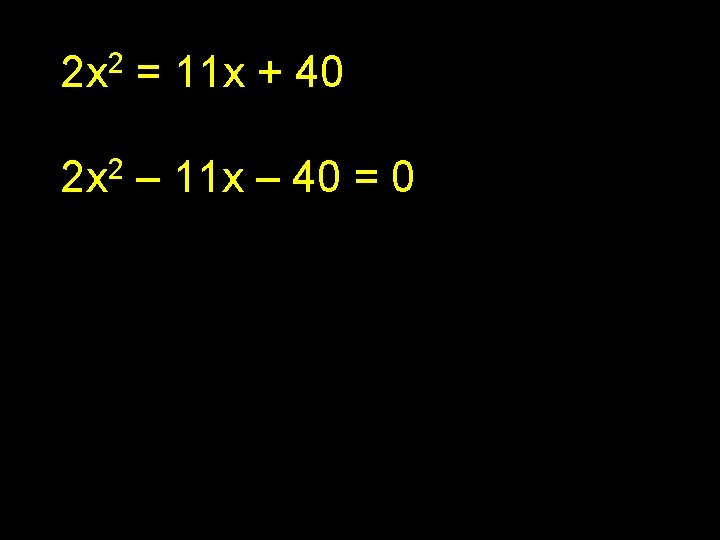2 x 2 = 11 x + 40 2 2 x – 11 x – 40 = 0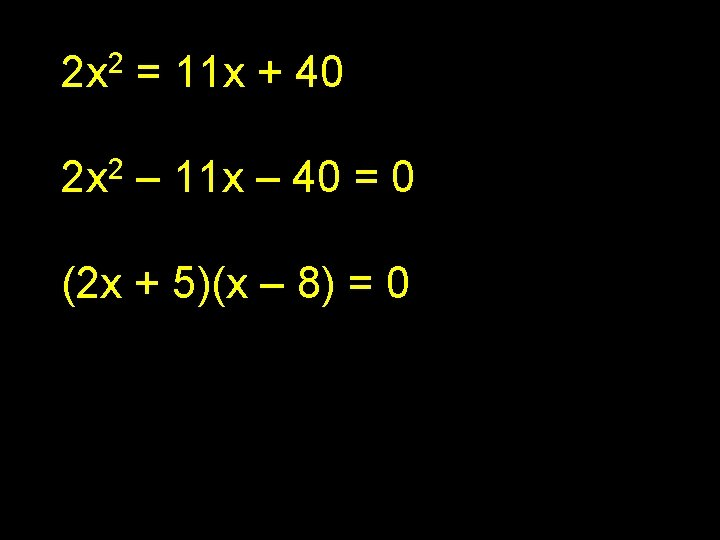2 x 2 = 11 x + 40 2 2 x – 11 x – 40 = 0 (2 x + 5)(x – 8) = 02 x 2 = 11 x + 40 2 2 x – 11 x – 40 = 0 (2 x + 5)(x – 8) = 0 x = -2/5 or x = 8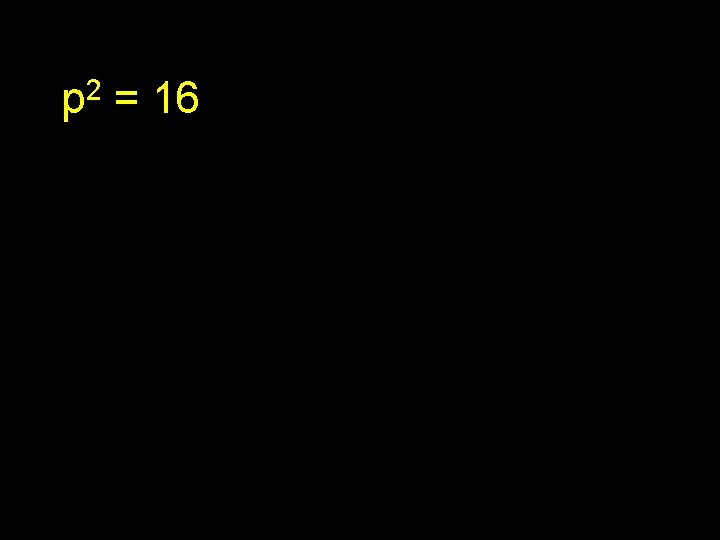2 p = 162 p = 16 p 2 – 16 = 02 p = 16 p 2 – 16 = 0 (p + 4)(p – 4) = 0 p = 4 or -4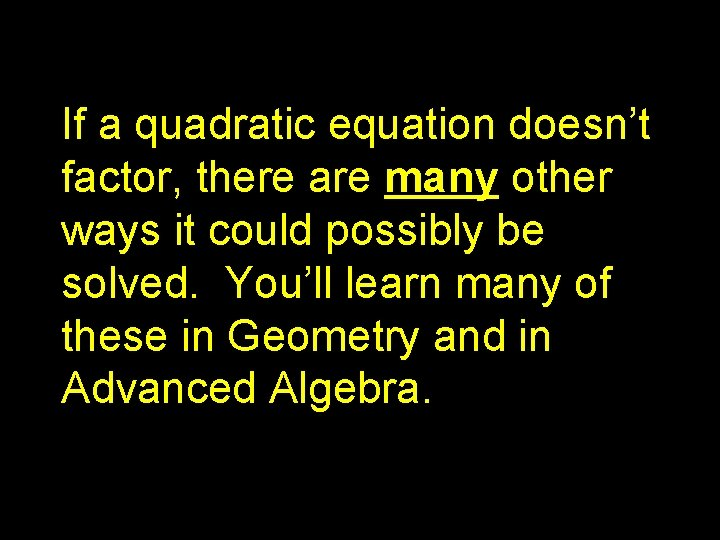If a quadratic equation doesn’t factor, there are many other ways it could possibly be solved. You’ll learn many of these in Geometry and in Advanced Algebra.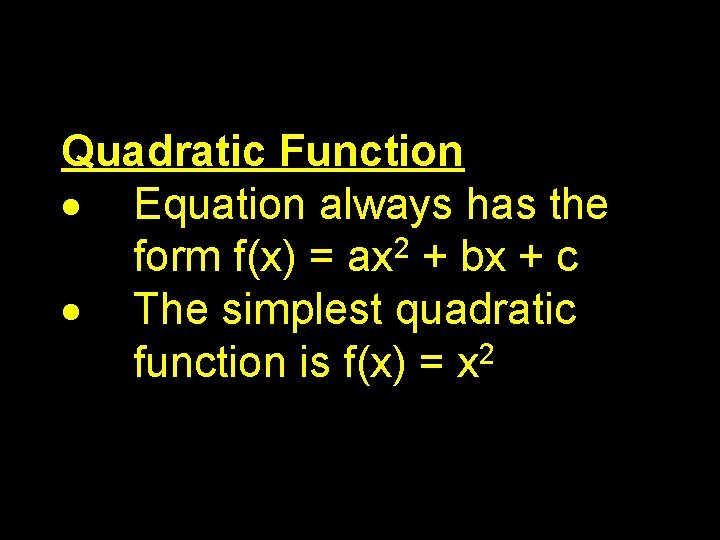Quadratic Function Equation always has the form f(x) = ax 2 + bx + c The simplest quadratic 2 function is f(x) = xGraph f(x) = x 2 Like the absolute value function, this gives the same answers for positive and negative numbers.However it is curved at the bottom rather than making a straight V-shape.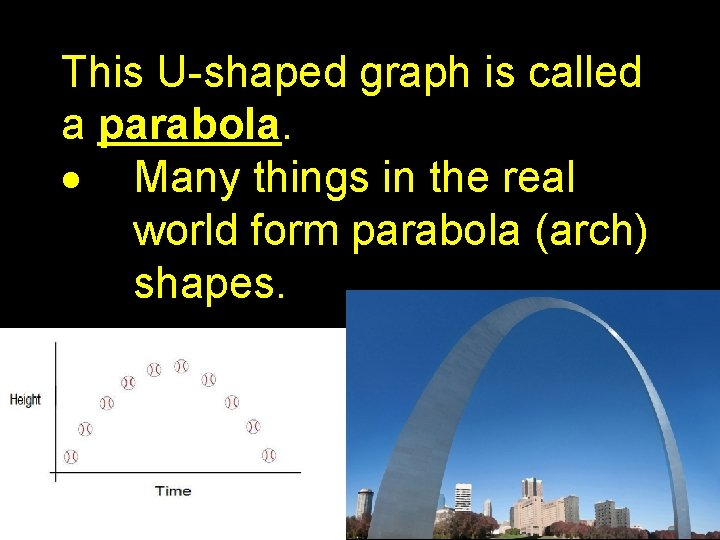This U-shaped graph is called a parabola. Many things in the real world form parabola (arch) shapes.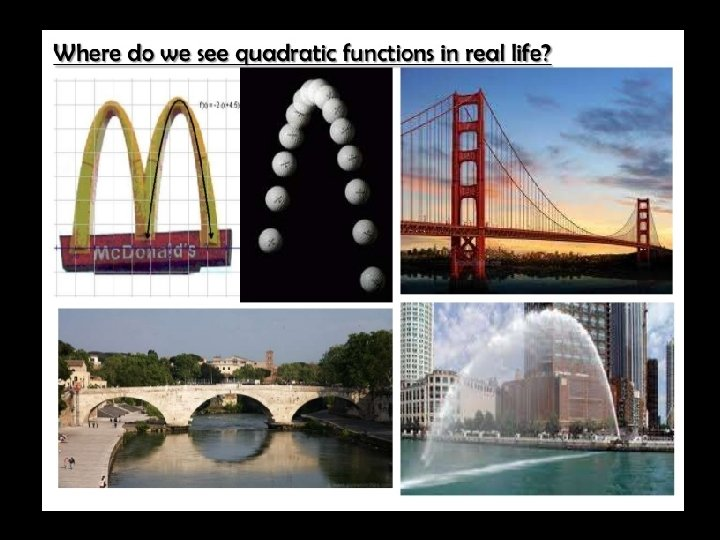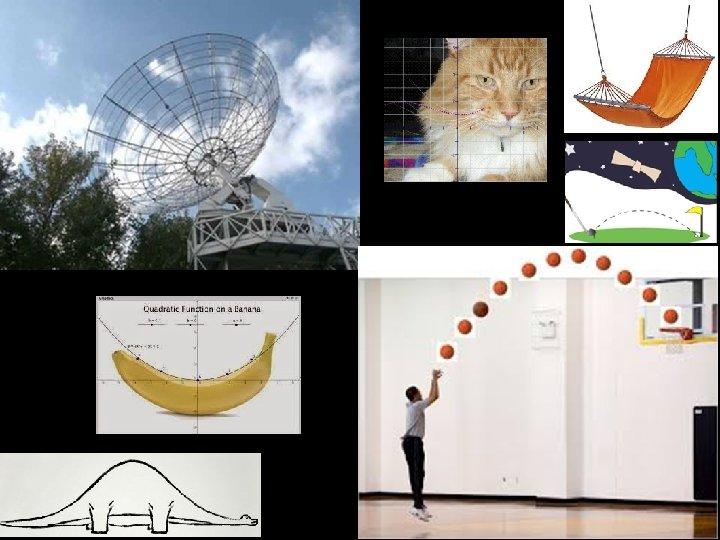Quadratic functions are used particularly in problems involving Movement and the force of gravity AreaWhat we usually care about with quadratic functions are the roots. These are the places where the function = 0 They can also be called “zeros” or “x-intercepts”.The roots of this quadratic function are -3 and 1. This is the same as the solutions to -x – 2 x + 3 = 0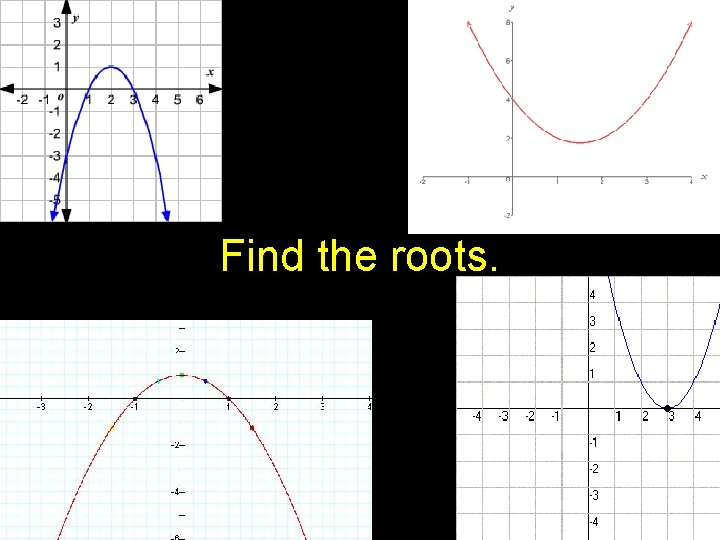Find the roots.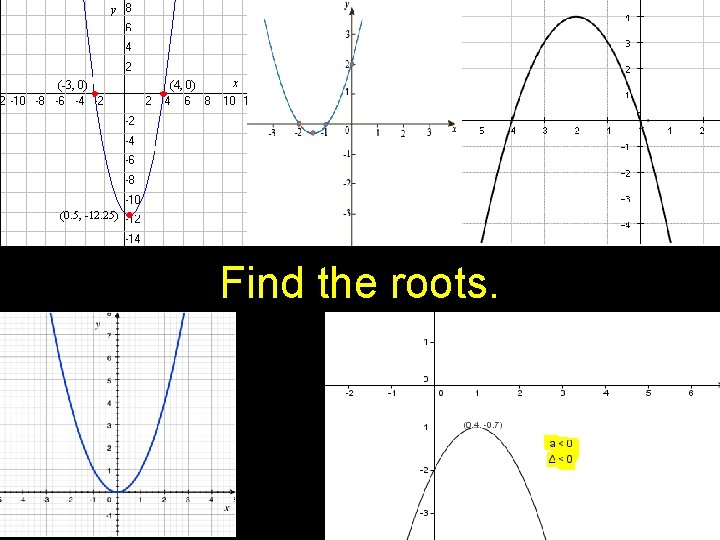Find the roots.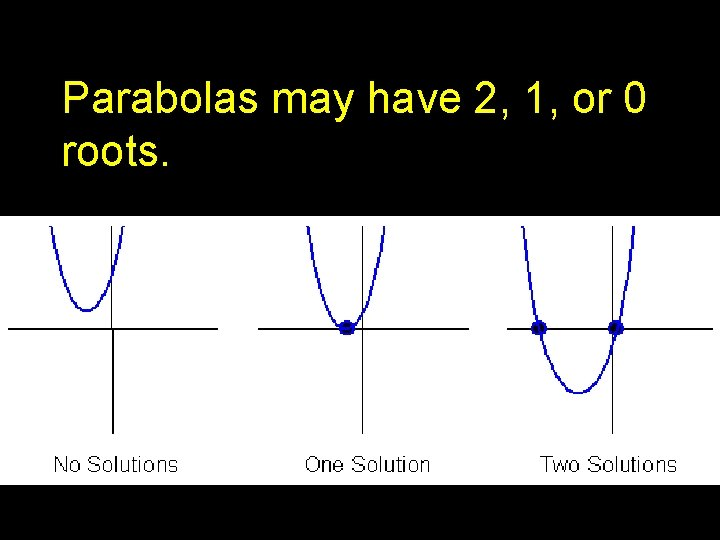Parabolas may have 2, 1, or 0 roots.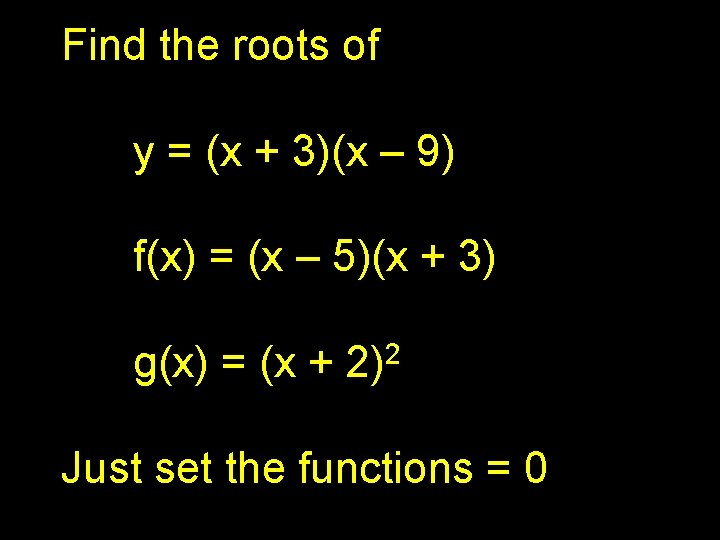Find the roots of y = (x + 3)(x – 9) f(x) = (x – 5)(x + 3) g(x) = (x + 2 2) Just set the functions = 0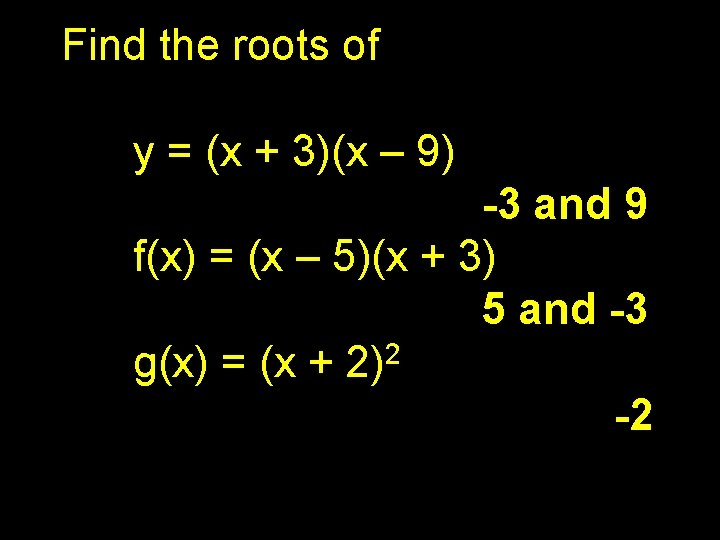Find the roots of y = (x + 3)(x – 9) -3 and 9 f(x) = (x – 5)(x + 3) 5 and -3 2 g(x) = (x + 2) -2Find the roots: f(x) = x 2 + 11 x + 28 y= 2 x – 11 x + 18 g(x) = x 2 + 1 x – 12Find the roots: f(x) = x 2 + 11 x + 28 (x + 7)(x + 4) -7 and -4 2 y = x – 11 x + 18 (x – 9)(x – 2) 9 and 2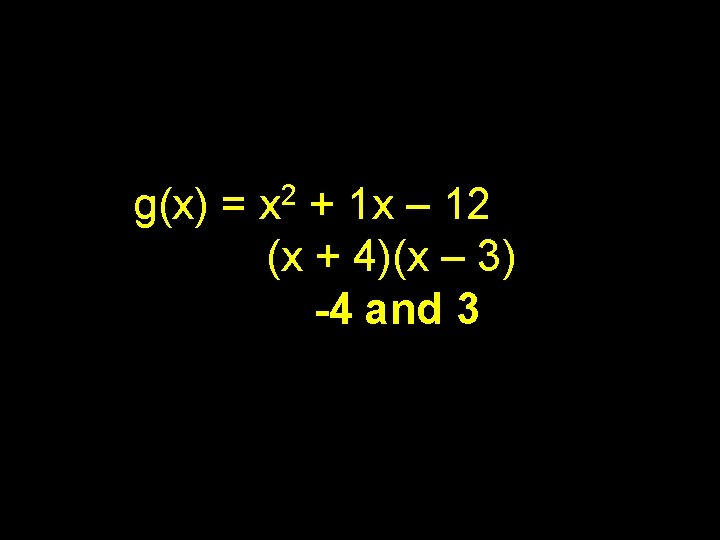g(x) = x 2 + 1 x – 12 (x + 4)(x – 3) -4 and 3Find the roots y = x(x – 8)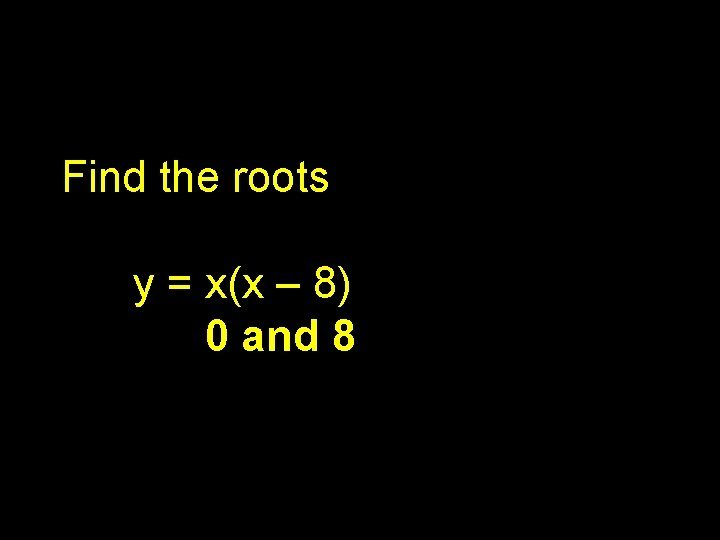Find the roots y = x(x – 8) 0 and 8